# ACT Math : How to find the length of the side of a 45/45/90 right isosceles triangle

## Example Questions

### Example Question #1 : How To Find The Length Of The Side Of A 45/45/90 Right Isosceles Triangle

A 44/45/90 triangle has a hypotenuse of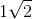. Find the length of one of its legs.

Cannot be determinedExplanation:

It's helpful to remember upon coming across a 45/45/90 triangle that it's a special right triangle. This means that its sides can easily be calculated by using a derived side ratio: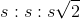Here,represents the length of one of the legs of the 45/45/90 triangle, and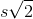represents the length of the triangle's hypotenuse. Two sides are denoted as congruent lengths () because this special triangle is actually an isosceles triangle. This goes back to the fact that two of its angles are congruent.

Therefore, using the side rules mentioned above, if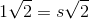, this problem can be resolved by solving for the value of: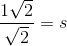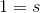Therefore, the length of one of the legs is 1.

### Example Question #2 : How To Find The Length Of The Side Of A 45/45/90 Right Isosceles Triangle

In a 45-45-90 triangle, if the hypothenuse is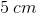long, what is a possible side length?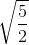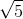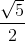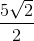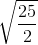Explanation:

If the hypotenuse of a 45-45-90 triangle is provided, its side length can only be one length, since the sides of all 45-45-90 triangles exist in a defined ratio of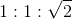, whererepresents the length of one of the triangle's legs andrepresents the length of the triangle's hypotenuse. Using this method, you can set up a proportion and solve for the length of one of the triangle's sides: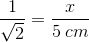Cross-multiply and solve for.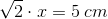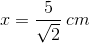Rationalize the denominator.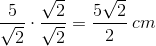You can also solve this problem using the Pythagorean Theorem.In a 45-45-90 triangle, the side legs will be equal, so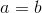. Substituteforand rewrite the formula.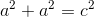Substitute the provided length of the hypothenuse and solve for.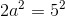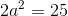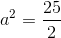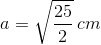While the answer looks a little different from the result of our first method of solving this problem, the two represent the same value, just written in different ways.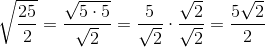### Example Question #11 : Isosceles Triangles

In a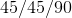triangle, if the length of the hypotenuse is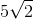, what is the perimeter?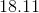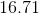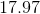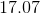Explanation:

1. Remember that this is a special right triangle where the ratio of the sides is: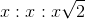In this case that makes it: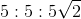2. Find the perimeter by adding the side lengths together: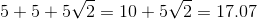### Example Question #4 : How To Find The Length Of The Side Of A 45/45/90 Right Isosceles Triangle

The height of atriangle is. What is the length of the hypotenuse?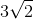Explanation:

Remember that this is a special right triangle where the ratio of the sides is:In this case that makes it: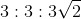Whereis the length of the hypotenuse.